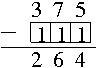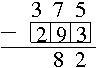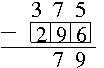# To regroup or not to regroup

Alignments to Content Standards: 4.NBT.B

Sometimes when we subtract one number from another number we "regroup," and sometimes we don't. For example, if we subtract 38 from 375, we can "regroup" by converting a ten to 10 ones:Find a 3-digit number to subtract from 375 so that:

1. You don't have to use regrouping.
2. You would naturally use regrouping from the tens to the ones place.
3. You would naturally use regrouping from the hundreds place to the tens place.
4. You would naturally use regrouping in all places.

In each case, explain how you chose your numbers and complete the problem.

## IM Commentary

In a typical subtraction task where students have learned and are using the standard US algorithm, we present a variety of problems that require regrouping in different places and ask students to solve them. This task presents an incomplete problem and asks students to choose numbers to subtract (subtrahends) so that the resulting problem requires different types of regrouping. This way students have to recognize the pattern and not just follow a memorized algorithm--in other words, they have to think about what happens in the subtraction process when we regroup. This task is appropriate to use after students have learned the standard US algorithm.

This is an instructional task; students would benefit from working in groups where they can each try different numbers and compare what they find. Once they have found numbers that work, they can explain to each other how they found them and why their choices require regrouping. Manipulatives like base ten blocks can be used to visualize and act out the regrouping process.

Giving a complete explanation is significantly harder than finding numbers that work in each case. But even if fourth graders shouldn't be expected to give as concise and complete an explanation as is listed in the solution, they can explore which digits in which place require regrouping and describe the general pattern.

## Solution

1. There are many possible answers. The important part is that the digit in the hundreds place is less than or equal to 3, the digit in the tens place is less than or equal to 7, and the digit in the ones place is less than or equal to 5. For example, 111 would work.Writing the problem in expanded form can help us see what is going on: \begin{align} 375-111 =& 300 + 70 + 5 - 100 - 10 - 1\\ =& 300-100 + 70 - 10 + 5 -1\\ =& 200 + 60 + 4\\ =& 264 \end{align}
2. There are many possible answers. The important part is that the digit in the ones place is greater than 5 while the digit in the hundreds place is less than or equal to 3 and the digit in the tens place is less than 7 (if it equals 7, then we will have to regroup from the hundreds place to the tens place after we regroup from the tens to the ones). For example, 266 would work.Writing the problem in expanded form can help us see what is going on: \begin{align} 375-266 =& 300 + 70 + 5 - 200 - 60 - 6\\ =& 300-200 + 70 - 60 + 5 - 6\\ =& 300-200 + 60 - 60 + 15 - 6\\ =& 100 + 9\\ =& 109 \end{align}
3. There are many possible answers. The important part is that the digit in the tens place is greater than 7 while the digit in the hundreds place is less than 3 and the digit in the ones place is less than or equal to 5. For example, 293 would work.4. There are many possible answers. The important part is that the digit in the ones place is greater than 5 and the digit in the tens place is greater than or equal to 7 while the digit in the hundreds place is less than 3 (if it equals 3, the difference will be negative, and students don't study signed numbers until 7th grade). For example, 296 would work.We already mentioned how we had to choose the number as part of the explanations above. To summarize and generalize (assuming we write the numbers stacked as shown to make the explanation easier):

• If all digits in the bottom number are less than or equal to the corresponding digits in the top number, we don't have to regroup to complete the problem.
• If the digit in the ones place on the bottom is greater than the digit in the ones place on the top, we will regroup from the tens place to the ones place.
• Similarly, if the digit in the tens place on the bottom is greater than the digit in the tens place on the top, we will regroup from the hundreds place to the tens place.
• To ensure regrouping in both places, the digit in the bottom number in the ones place has to be greater than the corresponding digit in the top number. The digit in the tens place of the bottom number has to be greater than or equal to the corresponding digit on the top.